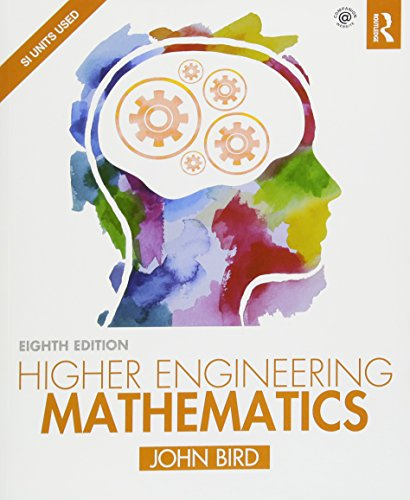# Higher Engineering Mathematics by John Bird

Out of Stock
\$42.99
+10% GST
N/A
Only 0 left

## Higher Engineering Mathematics Summary

### Higher Engineering Mathematics by John Bird

Now in its eighth edition, Higher Engineering Mathematics has helped thousands of students succeed in their exams. Theory is kept to a minimum, with the emphasis firmly placed on problem-solving skills, making this a thoroughly practical introduction to the advanced engineering mathematics that students need to master. The extensive and thorough topic coverage makes this an ideal text for upper-level vocational courses and for undergraduate degree courses. It is also supported by a fully updated companion website with resources for both students and lecturers. It has full solutions to all 2,000 further questions contained in the 277 practice exercises.

### Why buy from World of BooksOur excellent value books literally don't cost the earthFree delivery in AustraliaEvery used book bought is one saved from landfill

John Bird (BSc(Hons), CMath, CEng, CSci, FITE, FIMA, FCollT) is the former Head of Applied Electronics in the Faculty of Technology at Highbury College, Portsmouth, UK. More recently he has combined freelance lecturing and examining, and is the author of over 130 textbooks on engineering and mathematical subjects with worldwide sales of one million copies. He is currently lecturing at the Defence School of Marine Engineering in the Defence College of Technical Training at HMS Sultan, Gosport, Hampshire, UK.

Preface

Syllabus guidance

Section A Number and algebra

1 Algebra

2 Partial fractions

3 Logarithms

4 Exponential functions

5 Inequalities

6 Arithmetic and geometric progressions

7 The binomial series

8 Maclaurin's series

9 Solving equations by iterative methods

10 Binary, octal and hexadecimal numbers

11 Boolean algebra and logic circuits

Section B Geometry and trigonometry

12 Introduction to trigonometry

13 Cartesian and polar co-ordinates

14 The circle and its properties

15 Trigonometric waveforms

16 Hyperbolic functions

17 Trigonometric identities and equations

18 The relationship between trigonometric and

hyperbolic functions

19 Compound angles

Section C Graphs

20 Functions and their curves

21 Irregular areas, volumes and mean values of waveforms

Section D Complex numbers

22 Complex numbers

23 De Moivre's theorem

Section E Matrices and determinants

24 The theory of matrices and determinants

25 Applications of matrices and determinants

Section F Vector geometry 303

26 Vectors

27 Methods of adding alternating waveforms

28 Scalar and vector products

Section G Introduction to calculus

29 Methods of differentiation

30 Some applications of differentiation

31 Standard integration

32 Some applications of integration

33 Introduction to differential equations

Section H Further differential calculus

34 Differentiation of parametric equations

35 Differentiation of implicit functions

36 Logarithmic differentiation

37 Differentiation of hyperbolic functions

38 Differentiation of inverse trigonometric and hyperbolic functions

39 Partial differentiation

40 Total differential, rates of change and small changes

41 Maxima, minima and saddle points for functions of two variables

Section I Further integral calculus

42 Integration using algebraic substitutions

43 Integration using trigonometric and hyperbolic substitutions

44 Integration using partial fractions

45 The t = tan /2

46 Integration by parts

47 Reduction formulae

48 Double and triple integrals

49 Numerical integration

Section J Further differential equations

50 Homogeneous first order differential equations

51 Linear first order differential equations

52 Numerical methods for first order differential equations

53 First order differential equations of the form

54 First order differential equations of the form

55 Power series methods of solving ordinary differential equations

56 An introduction to partial differential equations

Section K Statistics and probability

57 Presentation of statistical data

58 Mean, median, mode and standard deviation

59 Probability

60 The binomial and Poisson distributions

61 The normal distribution

62 Linear correlation

63 Linear regression

64 Sampling and estimation theories

65 Significance testing

66 Chi-square and distribution-free tests

Section L Laplace transforms

67 Introduction to Laplace transforms

68 Properties of Laplace transforms

69 Inverse Laplace transforms

70 The Laplace transform of the Heaviside function

71 The solution of differential equations using Laplace transforms

72 The solution of simultaneous differential equations using Laplace transforms

Section M Fourier series

73 Fourier series for periodic functions of period 2

74 Fourier series for a non-periodic function over period 2

75 Even and odd functions and half-range Fourier series

76 Fourier series over any range

77 A numerical method of harmonic analysis

78 The complex or exponential form of a Fourier series

Section N Z-transforms

79 An introduction to z-transforms

Essential formulae

Index

GOR009240164
Higher Engineering Mathematics by John Bird
John Bird
Used - Very Good
Paperback
Taylor & Francis Ltd
2017-03-01
906
1138673579
9781138673571
N/A
Book picture is for illustrative purposes only, actual binding, cover or edition may vary.
This is a used book - there is no escaping the fact it has been read by someone else and it will show signs of wear and previous use. Overall we expect it to be in very good condition, but if you are not entirely satisfied please get in touch with us.